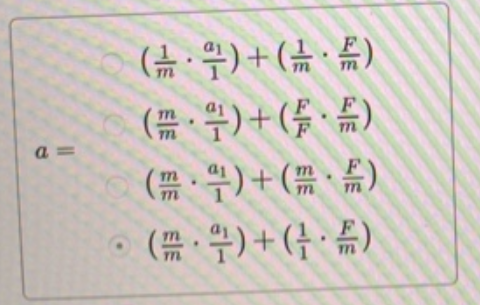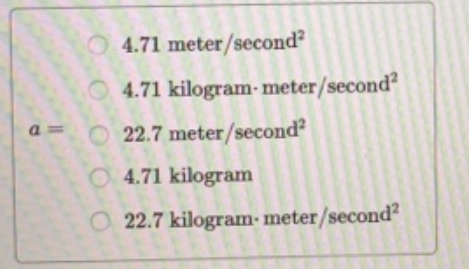# Problem: A student solving for the acceleration of an object has applied appropriate physics principles and obtained the expression  a = a1 + F/m  where  a1 = 3.00 m/s2,  F = 12.0 kg•m/s2 and  m = 7.00 kg.(a) First, which of the following is the correct step for obtaining a common denominator for the two fractions in the expression in solving for  a? (b) What is the numerical value of a?

###### FREE Expert Solution

(a)

79% (480 ratings)###### Problem Details

A student solving for the acceleration of an object has applied appropriate physics principles and obtained the expression  a = a1 + F/m  where  a1 = 3.00 m/s2,  F = 12.0 kg•m/s2 and  m = 7.00 kg.
(a) First, which of the following is the correct step for obtaining a common denominator for the two fractions in the expression in solving for  a(b) What is the numerical value of a?